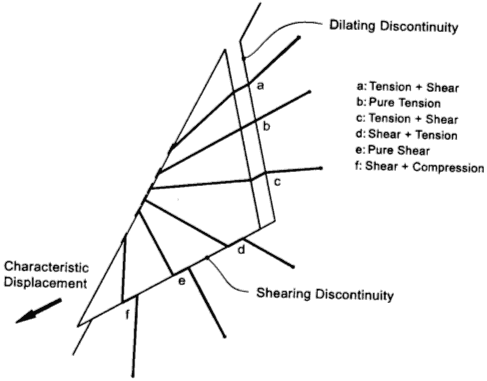# Bolt Support Force

The Bolt Support Force that is ultimately applied to a wedge and the orientation of the applied force (Tension or Shear) depend on the following factors:

Bolt Deformation Mode - The mode in which a bolt intersects a wedge failure plane. The deformation mode depends on whether the failure plane is a shear (sliding) plane or a dilating (opening) plane, and also on the orientation of the bolt with respect to the plane and the sliding direction. There are six possibilities considered, as illustrated in the figure below.Possible bolt deformation modes [Windsor, 1996]

Bolt Tensile Capacity - The maximum Tensile Force that can be supplied by the bolt is determined from the Bolt Force Diagram and the point at which a wedge plane intersects the bolt along its length. The Bolt Force Diagram is derived from the bolt properties (e.g., Tensile Capacity, Plate Capacity, Bond Strength) entered in the Bolt Properties dialog.

Bolt Orientation Efficiency - If considered, Bolt Orientation Efficiency is applied only to the Tensile Force. Bolt Orientation Efficiency can be toggled on or off, and there are three possible methods of computing the efficiency.

Bolt Shear Strength - Whether Shear Strength of the bolt is considered.

## Applied Bolt Support Force

The following table summarizes the possibilities of applied Bolt Support Force for all combinations of Bolt Deformation Mode, Bolt Orientation Efficiency (ON/OFF) and Bolt Shear Strength (ON/OFF).

 Bolt Deformation Mode Bolt Efficiency: Off Shear: Off Bolt Efficiency: On Shear: Off Bolt Efficiency: On Shear: On Bolt Efficiency: Off Shear: On A tensile tensile * eff tensile * eff tensile B tensile tensile * eff tensile * eff tensile C tensile tensile * eff tensile * eff tensile D tensile tensile * eff shear shear E tensile zero force shear shear F tensile zero force shear shear

Table 1: Summary of Bolt Support Force

#### NOTES:

• tensile is the Tensile Failure Capacity (without applying Bolt Orientation Efficiency) determined from the Bolt Force Diagram.
• eff is the Bolt Orientation Efficiency factor.
• shear is the bolt shear capacity, if the Bolt Shear Strength option is used.
• Modes A, B, C - If a bolt is in mode A, B or C, only a Tensile Force is applied in the direction of the bolt. The Tensile Force is multiplied by the Bolt Orientation Efficiency factor if Bolt Orientation Efficiency is being used.
• Mode D - If a bolt is in mode D, it can use either Tensile or Shear Force. If the Bolt Shear Strength option is ON, Shear Force is used. Shear Force is applied opposite to the sliding direction of the wedge. If the Bolt Shear Strength option is OFF, Tensile Force is used.
• Modes E, F - If a bolt is in mode E or F, it can use either Tensile, zero, or Shear Force, depending on the selection of Bolt Orientation Efficiency and Bolt Shear Strength.
• If both Bolt Orientation Efficiency and Bolt Shear Strength are turned OFF, the full Tensile Force is applied in the direction of the bolt, regardless of how the bolt intersects the wedge (i.e., even if the bolt is in a Shear Deformation Mode).

## Direction of Bolt Support Force

There are two possibilities for the direction of the applied Bolt Support Force (referring to Table 1):

Tensile - For all cases marked as tensile or tensile * eff, the direction of the applied Bolt Support Force is in the direction of the bolt.

NOTE: The direction is not affected by the Bolt Orientation Efficiency factor. The direction is always exactly in the direction of the bolt, even if the Bolt Orientation Efficiency factor is applied.

Shear - For all cases marked as shear (Bolt Shear Strength option is toggled ON), the direction of the applied Bolt Support Force is opposite to the sliding direction of the wedge.

A bolt can never apply both Tensile and Shear Support at the same time. The two cases are completely exclusive in the RocPlane implementation.